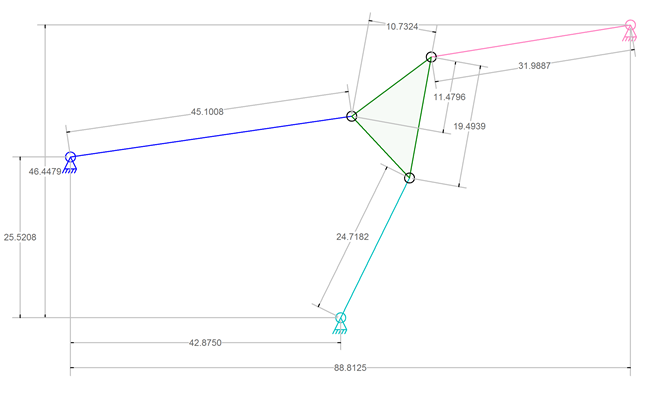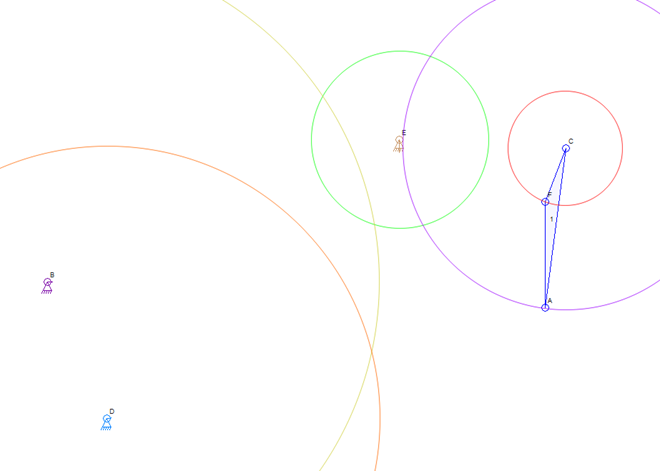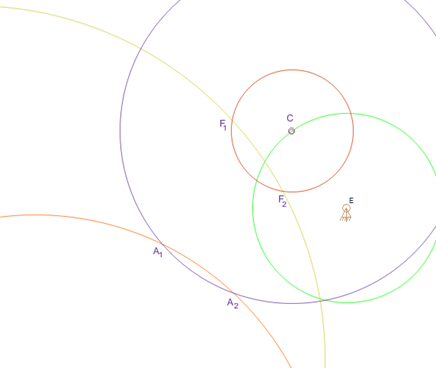In my previous blog post, about Computing the Position of a Triangle Based on Distances From External Points to Each Corner, I came up with a potential method of simulating a three link connection to a three connector link.Computing the Position of a Triangle Based on Distances From External Points to Each Corner

That process in the other blog post involved computing a curved path to represent a set of potential positions for a connector on the triangle link then finding where that path intersected a circle that defined another set of potential positions. The intersection points become the set of possible locations that would yield a valid physical position.

I present here another idea for determining the position of that triangle.Portion of Stephenson Valve Gear

Presented in the image above is a portion of the Stephenson valve gear for steam engines. This has the same properties as the first image where three fixed locations and three known lengths are used to position a triangle link.

My idea is to use another iterative algorithm but with only circles and angles used for determining potential positions of the triangle link.

The next image shows some circles drawn around the various parts of the mechanism. The links to the triangle link have been replaced by circles and a few circles have been added to the triangle link for reference when describing the algorithm.Circles Used For Analysis

The intersections of these various circles will be used to determine possible locations for the points of the triangle and an angle computation will be used to throw out bad positions.

The first step will be to move the red circle at C around the green circle at E. There is a way to exclude a large portion of the green circle but the whole thing is used for now. As the center of the red circle is moved around E along the green circle path, a distance is calculated to determine if the red circle will intersect the yellow circle around B. If the center of the red circle is not close enough then nothing is done and the red circle is moved again.

As soon as the red circle moving around the green circle intersects the yellow circle, There is now a possible location for the triangle link. The image blow shows one such possible position with the colored circles that have been moved so far.Possible Triangle Link Position

The next step is to figure out if the purple circle will reach the orange circle. With the circles in their respective positions in the image below, it is obvious that the triangle link cannot be positioned based on the red circle because the purple circle does not reach the orange circle.

These circles confuse me too. This is all done mathematically so far and only the circle center points and their radii are being used.Testing The Next Triangle Point

All of these test are checking to determine if the triangle link can be placed at the given location. The only point that is used as the basis for these tests is the center of the red circle moving along the green circle circumference.

After the red circle has moved some more, the complexity of the calculations increases. The purple circle radius is still used to determine if there is any reason to do further tests. The image below shows that the test passes and that a possible location has been found.A Potential Location

The next step is fairly simple. There are two possible places where the triangle link can be placed because there are two intersections of the red and yellow circles. There are also two possible locations for the other connector of the triangle link because there are two intersections of the purple and orange circles.

The distances between the points can be checked as well as the angle between the points. Whichever test is the most efficient will be used first followed by the other less efficient test. If the distances and one of the angles matches those in the triangle link then this is a valid location for it.

I have not refined the algorithm to the point of knowing the best way to handle very close calls. It is very easy to pass the valid solution without hitting it so some of the previous angles will be kept and any time the angle gets closer to being correct then starts getting further from correct, the algorithm will use smaller steps to try to narrow down the valid position of the triangle link.A Solution (Know Because of The Original Drawing)

This may lead to numerous solutions. Each solution is kept until a better solution is found that keeps the connectors closer to their previously simulated positions than the previous best solution.

I will post again about this if I try it and get good results. This is simpler than the four-bar idea from the previous blog post because there is only a change in the center points of the various test circles and no four-bar mechanism to simulate.